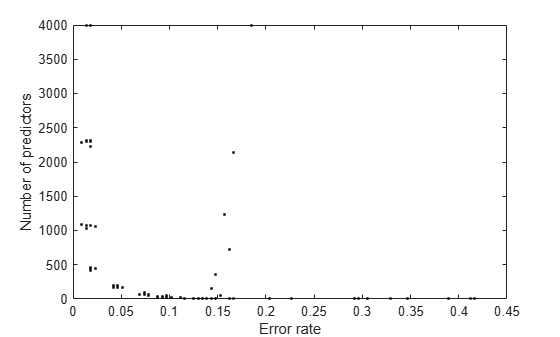# cvshrink

Cross-validate regularization of linear discriminant

## Syntax

```err = cvshrink(obj) [err,gamma] = cvshrink(obj) [err,gamma,delta] = cvshrink(obj) [err,gamma,delta,numpred] = cvshrink(obj) [err,...] = cvshrink(obj,Name,Value) ```

## Description

`err = cvshrink(obj)` returns a vector of cross-validated classification error values for differing values of the regularization parameter Gamma.

```[err,gamma] = cvshrink(obj)``` also returns the vector of Gamma values.

```[err,gamma,delta] = cvshrink(obj)``` also returns the vector of Delta values.

```[err,gamma,delta,numpred] = cvshrink(obj)``` returns the vector of number of nonzero predictors for each setting of the parameters Gamma and Delta.

`[err,...] = cvshrink(obj,Name,Value)` cross validates with additional options specified by one or more `Name,Value` pair arguments.

## Input Arguments

 `obj` Discriminant analysis classifier, produced using `fitcdiscr`.

### Name-Value Arguments

Specify optional pairs of arguments as `Name1=Value1,...,NameN=ValueN`, where `Name` is the argument name and `Value` is the corresponding value. Name-value arguments must appear after other arguments, but the order of the pairs does not matter.

Before R2021a, use commas to separate each name and value, and enclose `Name` in quotes.

 `delta` Scalar `delta` — `cvshrink` uses this value of `delta` with every value of `gamma` for regularization.Row vector `delta` — For each `i` and `j`, `cvshrink` uses `delta(j)` with `gamma(i)` for regularization.Matrix `delta` — The number of rows of `delta` must equal the number of elements in `gamma`. For each `i` and `j`, `cvshrink` uses `delta(i,j)` with `gamma(i)` for regularization.Default: `0` `gamma` Vector of Gamma values for cross-validation. Default: `0:0.1:1` `NumDelta` Number of Delta intervals for cross-validation. For every value of Gamma, `cvshrink` cross-validates the discriminant using `NumDelta + 1` values of Delta, uniformly spaced from zero to the maximal Delta at which all predictors are eliminated for this value of Gamma. If you set `delta`, `cvshrink` ignores `NumDelta`. Default: `0` `NumGamma` Number of Gamma intervals for cross-validation. `cvshrink` cross-validates the discriminant using `NumGamma + 1` values of Gamma, uniformly spaced from `MinGamma` to `1`. If you set `gamma`, `cvshrink` ignores `NumGamma`. Default: `10` `verbose` Verbosity level, an integer from `0` to `2`. Higher values give more progress messages. Default: `0`

## Output Arguments

 `err` Numeric vector or matrix of errors. `err` is the misclassification error rate, meaning the average fraction of misclassified data over all folds. If `delta` is a scalar (default), `err(i)` is the misclassification error rate for `obj` regularized with `gamma(i)`.If `delta` is a vector, `err(i,j)` is the misclassification error rate for `obj` regularized with `gamma(i)` and `delta(j)`.If `delta` is a matrix, `err(i,j)` is the misclassification error rate for `obj` regularized with `gamma(i)` and `delta(i,j)`. `gamma` Vector of Gamma values used for regularization. See Gamma and Delta. `delta` Vector or matrix of Delta values used for regularization. See Gamma and Delta. If you give a scalar for the `delta` name-value pair, the output `delta` is a row vector the same size as `gamma`, with entries equal to the input scalar.If you give a row vector for the `delta` name-value pair, the output `delta` is a matrix with the same number of columns as the row vector, and with the number of rows equal to the number of elements of `gamma`. The output `delta(i,j)` is equal to the input `delta(j)`.If you give a matrix for the `delta` name-value pair, the output `delta` is the same as the input matrix. The number of rows of `delta` must equal the number of elements in `gamma`. `numpred` Numeric vector or matrix containing the number of predictors in the model at various regularizations. `numpred` has the same size as `err`. If `delta` is a scalar (default), `numpred(i)` is the number of predictors for `obj` regularized with `gamma(i)` and `delta`.If `delta` is a vector, `numpred(i,j)` is the number of predictors for `obj` regularized with `gamma(i)` and `delta(j)`.If `delta` is a matrix, `numpred(i,j)` is the number of predictors for `obj` regularized with `gamma(i)` and `delta(i,j)`.

## Examples

expand all

Regularize a discriminant analysis classifier, and view the tradeoff between the number of predictors in the model and the classification accuracy.

Create a linear discriminant analysis classifier for the `ovariancancer` data. Set the `SaveMemory` and `FillCoeffs` options to keep the resulting model reasonably small.

```load ovariancancer obj = fitcdiscr(obs,grp,... 'SaveMemory','on','FillCoeffs','off');```

Use 10 levels of `Gamma` and 10 levels of `Delta` to search for good parameters. This search is time-consuming. Set `Verbose` to `1` to view the progress.

```rng('default') % for reproducibility [err,gamma,delta,numpred] = cvshrink(obj,... 'NumGamma',9,'NumDelta',9,'Verbose',1);```
```Done building cross-validated model. Processing Gamma step 1 out of 10. Processing Gamma step 2 out of 10. Processing Gamma step 3 out of 10. Processing Gamma step 4 out of 10. Processing Gamma step 5 out of 10. Processing Gamma step 6 out of 10. Processing Gamma step 7 out of 10. Processing Gamma step 8 out of 10. Processing Gamma step 9 out of 10. Processing Gamma step 10 out of 10. ```

Plot the classification error rate against the number of predictors.

```plot(err,numpred,'k.') xlabel('Error rate'); ylabel('Number of predictors');```• Examine the `err` and `numpred` outputs to see the tradeoff between cross-validated error and number of predictors. When you find a satisfactory point, set the corresponding `gamma` and `delta` properties in the model using dot notation. For example, if `(i,j)` is the location of the satisfactory point, set
```obj.Gamma = gamma(i); obj.Delta = delta(i,j);```